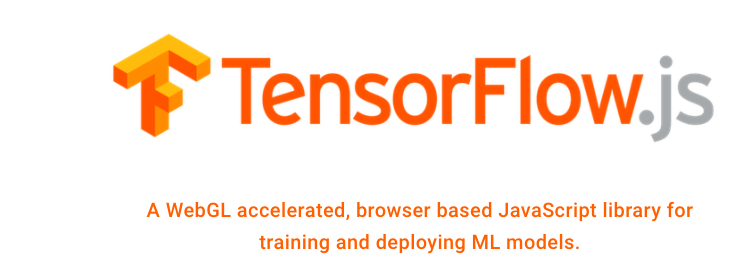# The Possibilities Around Streaming Data And TensorFlow.js In The BrowserWe are exploring the possibilities with streaming data and TensorFlow, a WebGL accelerated, browser based JavaScript library for training and deploying ML models. The new project opens up a whole new world of machine learning, client-side in the browser, and we wanted to begin thinking more about how Server-Sent Events (SSE) can be used to feed rich data and content into machine learning models operating within the browser. The project is just getting started, so the working examples of what it does are just getting started also, but we would like to begin with profiling what TensorFlow.js does.

TensorFlow.js moves machine learning into the browser, allowing us to:

Develop ML in the Browser – Use flexible and intuitive APIs to build and train models from scratch using the low-level JavaScript linear algebra library or the high-level layers API
Run Existing Models – Use TensorFlow.js model converters to run pre-existing TensorFlow models right in the browser.
Retrain Existing Models – Retrain pre-existing ML models using sensor data connected to the browser, or other client-side data.

TensorFlow.js delivers an open source solution that provide the logic and scripts that combine two packages:

TensorFlow.js Core – a flexible low-level API, formerly known as deeplearn.js.
TensorFlow.js Layers – a high-level API which implements functionality similar to Keras.

Most of the working examples right now of TensorFlow.js are multimedia based, working with images, and delivering games, however the default example below shows how to take some sample data, then run the model using the data, then use the model to do inference on a data point the model hasn’t seen before.

``````
<html>
<script src="https://cdn.jsdelivr.net/npm/@tensorflow/tfjs@0.9.0"> </script>
<script>

// Define a model for linear regression.
const model = tf.sequential();

// Prepare the model for training: Specify the loss and the optimizer.
model.compile({loss: 'meanSquaredError', optimizer: 'sgd'});

// Generate some synthetic data for training.
const xs = tf.tensor2d([1, 2, 3, 4], [4, 1]);
const ys = tf.tensor2d([1, 3, 5, 7], [4, 1]);

// Train the model using the data.
model.fit(xs, ys).then(() => {
// Use the model to do inference on a data point the model hasn't seen before:
// Open the browser devtools to see the output
model.predict(tf.tensor2d(, [1, 1])).print();
});
</script>